# Introduction to Heap Data Structure

### What is a Heap?

A heap is a complete binary tree structure where each element satisfies a heap property. In a complete binary tree, all levels are full except the last level, i.e., nodes in all levels except the last level will have two children. The last level will be filled from the left. Here, each heap node stores a value key, which defines the relative position of that node inside the heap.

Visualization of complete binary tree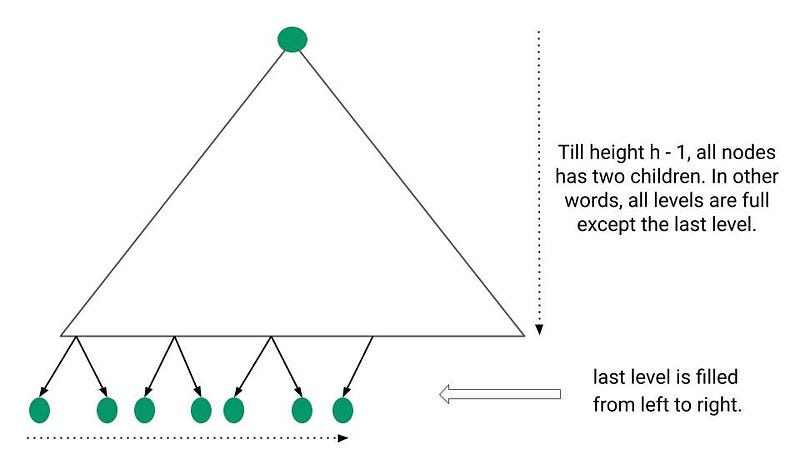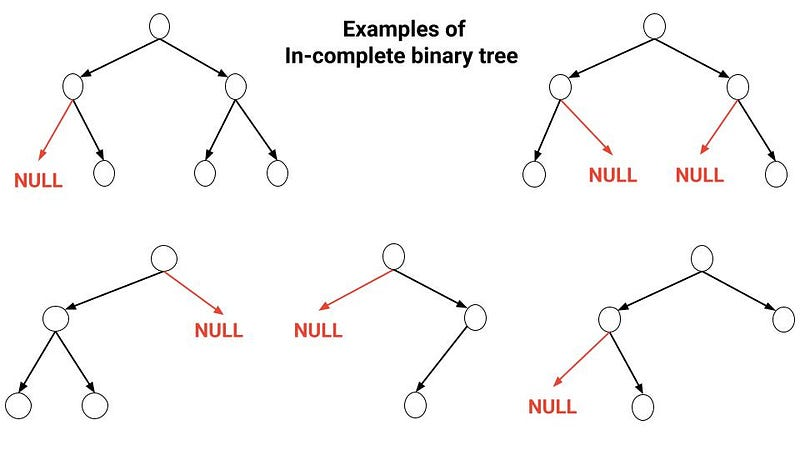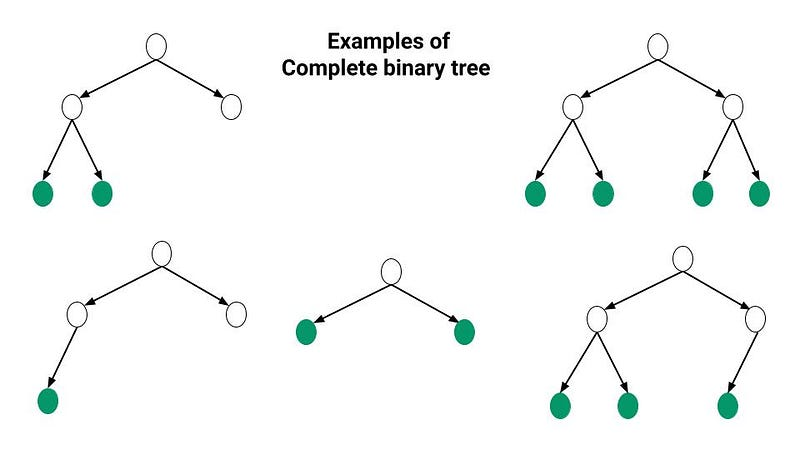There are two types of heaps as follows:

### Max-Heap

All elements in this heap satisfy the property that the key of the parent node is greater than or equal to the keys of its child nodes i.e. key of a node >= key of its children. So moving up from any node, we get a nondecreasing sequence of keys, and moving down from any node, we get a nonincreasing sequence of keys. In particular: The largest key in a max-heap is found at the root.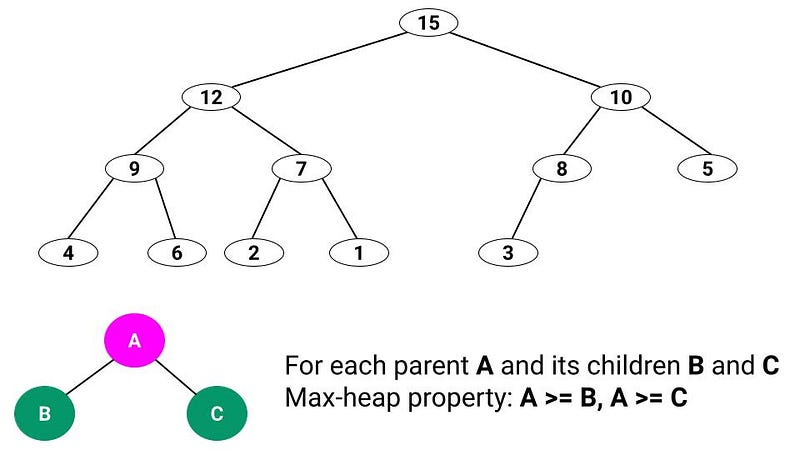### Min-Heap

All elements in this heap satisfy the property that the key of the parent node is less than or equal to the keys of its child nodes i.e. key of a node <= key of its children. So moving up from any node, we get a nonincreasing sequence of keys and moving down from any node, we get a nondecreasing sequence of keys. In particular: The largest key in a max-heap is found at the root.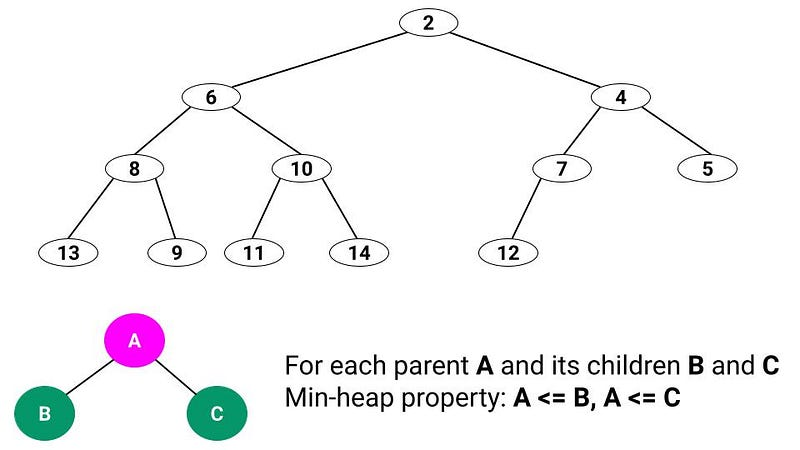### Special notes related to the Heap

• A binary heap is a binary tree that satisfies two properties: (1) Shape property: all levels, except the last level, are fully filled and the last level is filled from left to right (2) Heap property
• Level order traversal of the heap will give the order in which elements are filled in the array.
• Heap is a complete tree structure, so we define the height of a node in a heap as the number of edges on the longest path from the node to a leaf.
• We define the height of the heap to be the height of its root. Since a heap of n elements is based on a complete binary tree, its height is O(logn).
• In the worst case, we shall see that the basic operations on heaps run in time proportional to the tree's height and thus take O(logn) time.

### Array implementation of Heap

A binary heap can be represented using an array where the indices of the array capture the parent-child relationship. Suppose A[] be a heap array of size n:

• The root of the binary heap is stored at A.
• Given element A[i], the children of this element are stored in A[2i + 1] and A[2i + 2], if they exist.
• The left child of i denoted as left(i) = A[2i + 1], if 2i + 1 < n
• The right child of i denoted as right(i) = A[2i + 2], if 2i + 2 < n
• The parent of A[i] is stored in A[(i−1)/2].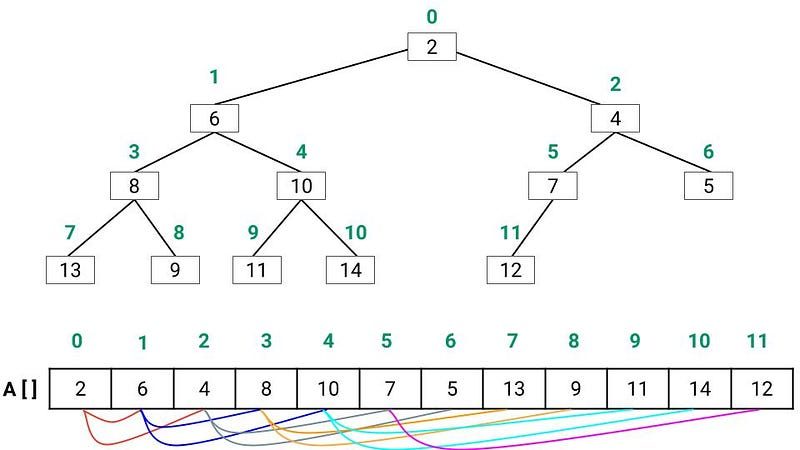Note: In most programming languages, these operations can be implemented efficiently using bitwise operators. Therefore, an array representation is a space-efficient approach as we don’t need to store extra 3 pointers per node in the heap.

• Thus, for a max-heap, we can say that A[i] ≥ A[2i + 1] and A[i] ≥ A[2*i + 2], where (2*i + 1) and (2i + 2) are < n. As we know, the key of each node in a max-heap is greater than the key of its children, hence, the maximum key in the heap will be stored at the root, that is, at A.
• Similarly, min-heap will satisfy the property that for any index i, A[i] ≤ A[2i + 1] and A[i] ≤ A[2*i + 2], where (2*i + 1) and (2i + 2) are < n. Thus, for a min-heap, the minimum element will be at the root of the heap and thus, at A.

### Operations supported by Max-Heap

Various operations supported by max heap is described below on a high level and will be covered in more detail in subsequent articles:

• maxHeapify(A[], i): It is a method to rearrange the elements of the heap in order to maintain the heap property. This process is required when a certain node at index i causes an imbalance in the heap due to some operation on that node.
• buildMaxHeap(A[]): We can use the procedure to convert an input array into a max-heap.
• findMax(heap[]): This operation returns the maximum value in the heap and its time complexity is O(1) as it just needs to return A.
• extractMax(A[]): This operation removes the maximum element from the heap and returns it. The time complexity of this operation is O(logn) as we replace A with A[n-1] — the last element of the heap, and then do some operations to maintain the max-heap property.
• increaseKey(A[], i, v): This operation increases the value at index i in the array to value v. This operation is only valid if A[i] ≤ v, that is the new value is greater than the existing value at index i. This ensures that the subtree rooted at index i is still a max-heap. The complexity of this operation is O(logn) as after increasing the key at index i, the max-heap property of Parent(i) might be violated, and we might need to perform some operations to restore it.
• insertKey(A[], v): This operation inserts the element v in a heap, and its complexity is O(lg n). To implement this operation, we add an element at the end of the heap (at A[n-1]) and then perform some operations to restore the heap property.
• deleteKey(A[], i): This operation is used to delete an element at index i, and the complexity of this operation is O(logn). To delete any element, we can replace it with the last element of the heap, and then again perform operations to restore the heap property in case it is violated.

In a similar fashion min-heap support these operations:

• minHeapify(A[], i)
• buildMinHeap(A[])
• findMin(A[])
• extractMin(A[])
• decreaseKey(A[], i, v)
• insertKey(A[], v)
• deleteKey(A[], i).

### Popular applications of Heap

• Heaps are used to efficiently implement a priority queue, an important data structure in computer science. One of the applications of priority queues is in process scheduling in operating systems.
• Heaps are used by the Heapsort Algorithm, which is one of the fastest sorting algorithms known. Its complexity is O(nlogn).
• Heaps are also used in the efficient implementations of algorithms like Dijkstra’s shortest-path algorithm, where we need to pick the node closest to a given node. If distances to all the nodes are stored in a heap then the closest node can be extracted efficiently using min-heap.
• Heaps provide an efficient way to get the kth smallest or largest element in an array.

### Critical ideas to think!

• How to calculate child and parent nodes of any node at index i using bitwise operations?
• How can we implement a heap using pointers?
• What will be the advantages of using pointers to implement heap? In which cases should we prefer pointer implementation over array implementation?
• The minimum number of elements in a heap of height h is 2^h.
• The maximum number of elements in a heap of height h is 2^(h+1) - 1.
• Where the smallest element resides in a max-heap might, assuming that all elements are distinct?
• Is an array that is in sorted order a min-heap?
• With the array representation for storing an n-element heap, the leaves are the nodes indexed by n/2, n/2 + 1, n/2 + 2 ..... n-1.

Enjoy learning, Enjoy Algorithms!

Get well-designed application and interview centirc content on ds-algorithms, machine learning, system design and oops. Content will be delivered weekly.